Friday, February 3

Today is devoted to an investigation of space curves. The geometry of these curves, as seen from the point of view of calculus (called "differential geometry of space curves") is a subject which originated in the 1800's. The material presented here was stated in about 1850-1870. It has within the last few decades become very useful in a number of applications: robotics, material science (structure of fibers), and biochemistry (the geometry involving the structure of big molecules such as DNA).

I'll carry along is a right circular helix as a basic example.

x(t)=a cos(t)
y(t)=a sin(t)
z(t)=b t
The quantities a and b are supposed to be positive real numbers. This helix has the z-axis as axis of symmetry. It lies "above" the circle with radius a and center (0,0) in the (x,y)-plane. The distance between two loops of the helix is 2Pi b.

If r(t)=x(t)i+y(t)j+z(t)k (the position vector), then r'(t)=x'(t)i+y'(t)j+z'(t)k=(ds/dt)T(t) is called the velocity vector. Here T(t) is called the unit tangent vector and is a unit vector in the direction of r'(t). ds/dt is the speed, and is sqrt(x'(t)2+y'(t)2+z'(t)2), the length of r'(t). We use ds/dt also to convert derivatives with respect to t to derivatives with respect to s, as last time (the Chain Rule).

Since T(t)·T(t)=1 differentiation together with commutativity of dot product gives 2T'(t)·T(t)=0, so T'(t) and T(t) are perpendicular. In fact, we are interested in dT/ds, which is the same as (1/(ds/dt))T'(t) (it is usually easier to compute T'(t) directly, however, and "compensate" by multiplying by the factor 1/(ds/dt)). Any non-zero(!) vector is equal to the product of its magnitude times a unit vector in its direction. For dT/ds, the magnitude is defined to be the curvature, and the unit vector is defined to be the unit normal N(t). This essentially coincides with what was done last time, when curvature was defined to be d(theta)/ds.

I remarked in one class that the two-dimensional version of curvature (the d(theta)/dt) could be negative. there could be problems if dT/ds is zero or if d(theta)/ds was negative (example: look at how T and N change for y=x3 as x goes from less than 0 to greater than 0). I will just ignore that here.

For the helix, we computed ds/dt=(sqrt(a2+b2)) and T(t)=(1/sqrt(a2+b2))(-a sint(t)i+a cos(t)j +bk) and also N(t)=-cos(t)i-sin(t)j. We noted that N(t) in this case always pointed directly towards the axis of symmetry. We also got a formula for curvature: kappa=a/(a2+b2). This is a constant, and partly you should be able to convince yourself that curvature is a constant in this case because each piece of this curve is congruent to each other piece of the curve: just move the helix along itself by sort of screwing it up or down as needed. We will also check the answer with some examples soon.

We "complete" T and N to what is called a 3-dimensional frame by defining the binormal B(t) to be the cross-product of T(t) and N(t). Since T(t) and N(t) are orthogonal unit vectors, B(t) is a unit vector orthogonal to both of them. This needs some thinking about, using properties of cross-product: certainly B(t) is perpendicular to each of its factors, and the size of B(t) is the area of the parellelogram determined by T(t) and N(t), and this parellelogram is a square one unit on each side.

How does B(t) change? Since B(t)·B(t)=1, differentiation results in 2B'(t)·B(t)=0, so B'(t) is orthogonal to B(t). But differentiation of B(t)=T(t)xN(T) results in B'(t)=T'(t)xN(t)+T(t)xN'(t). Since T'(t) is parallel to N(t) (because of our definition of N(t)!), the first product is 0 (another property of cross-product!) so that B'(t) is a cross-product of T(t) with something. Therefore B'(t) is also perpendicular to T(t). Well: B'(t) is perpendicular to both T(t) and B(t), and therefore, since only one direction is left, B'(t) must be a scalar multiple of N(t). The final important definition here for space curves is: dB/ds is a product of a scalar and N(t). The scalar is -tau. That is supposed to be the Greek letter tau, and the minus sign is put there so that examples (the most important is coming up!) will work out better. This quantity is called torsion, and is a measure of "twisting", how much a curve twists out of a plane (the particular plane is the plane determined by T and N).

If a space curve does lie in a plane, and if everything is nice and continuous, then B will always point in one direction (there are only two choices for B, "up" and "down" relative to the plane, and by continuity only one will be used) so that the torsion is 0 since B doesn't change. The converse implication (not verified here!) is also true: if torsion is always 0, then the curve must lie in a plane!

For our example, we computed B(t) by directly computing the cross-product of T(t) and N(t). We got (I think!) (1/(1/sqrt(a2+b2))(b sin(t)i-b cos(t)j+a k) for B(t). This can be "checked" in several ways. First, that the candidate for B(t) has unit length, and then, that B(t) is orthogonal to both T(t) and N(t). This candidate passes those tests. Then we took d/dt of this B(t) and multiplied it by 1/(ds/dt)=(1/sqrt(a2+b2)). The result was (b/(a2+b2))(cos(t)i+sin(t)j). Checking all the minus signs (one in the definition of torsion and one in the result of N(t)) shows that here torsion is (b/(a2+b2)).

Looking at the extreme values of a and b in this expression (a, b separately big and small) is not as revealing and/or as useful as with curvature, since a "feeling" for torsion isn't as immediate.
Then I looked at dN/ds, using the expression N=BxT. The result, after using the product rule carefully (remember that this product is not commutative!) is (dB/ds)xT+Bx(dT/ds) which, by the earlier equations, is -tauNxT+kappaBxN which is tauB-kappaT. So we have the following equations, called the Frenet-Serret equations (also called the Darboux equations in mechanics):

dT/ds =   0    + kappaN +  0
dN/ds =-kappaT +   0    + tauB
db/ds =   0      -tauN  +  0
This is a collection of 3 3-dimensional vector equations, so it is a collection of 9 scalar differential equations. The remarkable fact is that if an initial point is specified for the curve, and an initial "frame" for the Frenet frame of T, N, and B, and if the curvature and torsion are specified, then the solutions to the differential equations above give exactly one curve. All the information about the curve is contained in the equations. So, for example, the motion of an airplane or a robot arm or the (geometric) structure of a long molecule are, in some sense, completely specified by kappa and tau. Of course, this doesn't tell you really how to effectively control something so it moves or twists the way it is "supposed" to. The idea that the Frenet frame "evolves" in time, governed by the differential equations above, is useful.

Here are some pictures of various helices produced by Maple (the plural of "helix" is "helices").
The pictures below were produced using the command spacecurve([a*cos(t),a*sin(t),b*t],t=0..6*Pi,axes=normal,color=black,thickness=2,scaling=constrained,numpoints=180); The procedure spacecurve is loaded as part of plots using the command with(plots);. I used the option scaling=constrained in order to "force" Maple to display the three curves with similar spacing on the axes. Otherwise the x and y variables would be much altered in each image. I hope that these pictures give some idea of what the curvature and torsion represent.
Some helices: x=a cos(t) & y=a sin(t) & z=bt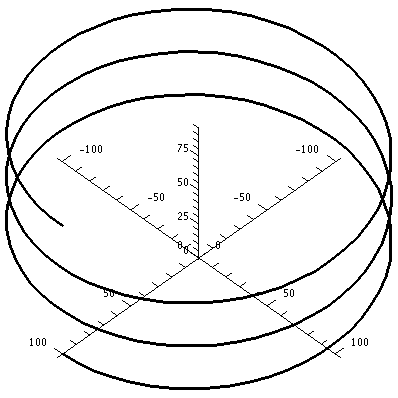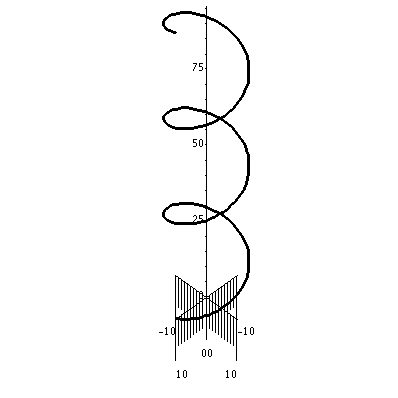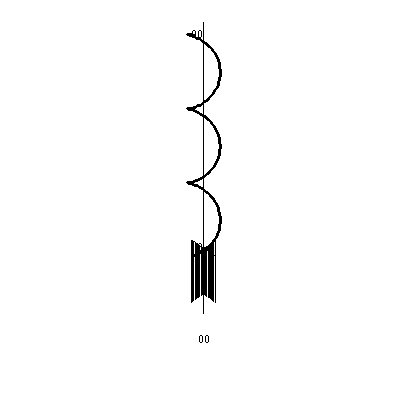a=100 & b=5
kappa=.01 & tau=.0005
a=10 & b=10
kappa=.05 & tau=.05
a=5 & b=100
kappa=.0005 & tau=.01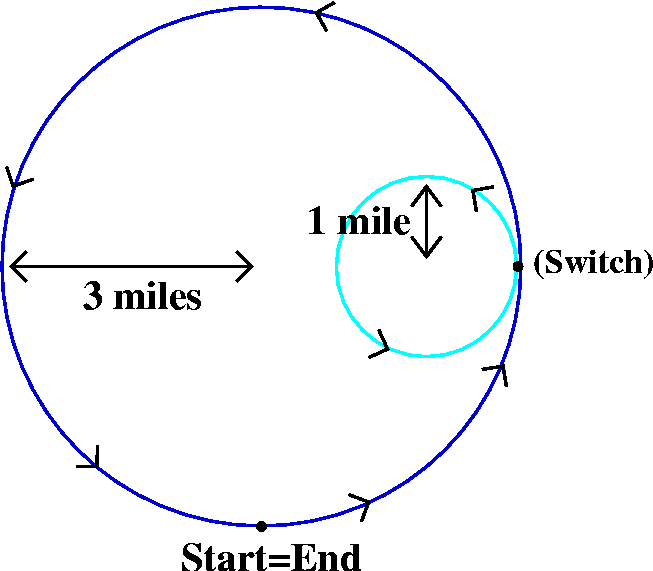QotD
Another racetrack: this racetrack consists, as nearly as possible, of two circles, one with radius 1 and one with radius 3. They are tangent at a point, and a quarter way counterclockwise around the big circle is the starting point which coincides with the end. This racetrack is what's called a closed curve. After the start, at the point of tangency, the racecars switch to the inside curve, and then go back to the outside curve when they complete a loop of the inside. I asked for a graph of the curvature. Please notice that I requested the graph of the curvature be "smooth".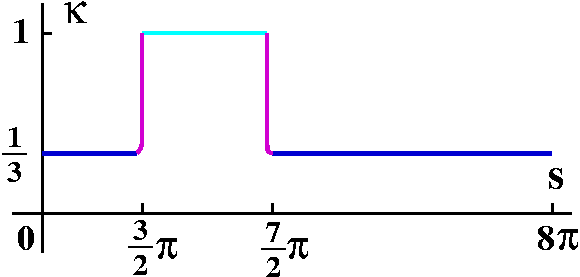I attempted to be "correct" both qualitatively and quantitatively in this graph. The magenta curves are my attempts to draw smooth transitions between the curvatures of the circles.

Right now I am having "fun" trying to design an effective Maple problem with curvature for the class.

Tuesday, January 31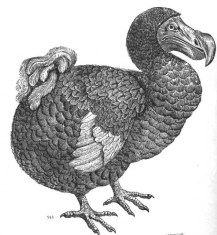A word from the management ...
The instructor gave a rather terrible presentation Tuesday morning in front of the students of sections 5, 6, and 7. An apology is due to them. He made at least one serious conceptual error, and several pedagogical errors. The notes below are mostly from the later lecture, given to students in sections 8, 9, and 10. While that lecture was not perfect, it went somewhat better than the earlier lecture. The notes below are mostly, therefore, from the second lecture.

Arc length
If r(t)=<12t,8t3/2,3t2<, problem #6 of section 13.2 asks for the length of the curve from t=0 to t=1. This is a typical artificial problem in a textbook. We know that r´(t)=<12,8(3/2)t1/2,6t< so that the speed is |r´(t)|=sqrt{122+122t+36t2}. Now we "remember" that distance=rate·time, and that the magnitude of the velocity vector is ds/dt, the speed. So the total distance traveled is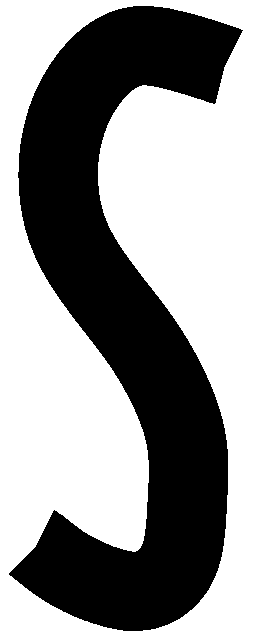01sqrt{144+144t+36t2} dt. Now look at the coincidences. We can pull out the 36 from under the square root, and the result is 601sqrt{4+4t+t2} dt, and, what a coincidence, 4+4t+t2 is (2+t)2 and the integral becomes 601(2+t) dt=6(2t+(1/2)t2]01 and this is 6[2+(1/2)].

More realistically ...
Almost every combination of two or three functions, even rather "simple" functions that you try to use as a position vector, will yield something that can't be antidifferentiated in terms of familiar functions. For example, look at the following mess:

> a:=t->t^3;
3
a := t -> t

> b:=t->cos(t);
b := t -> cos(t)

> c:=t->exp(t);
c := t -> exp(t)

> int(sqrt(a(t)^2+b(t)^2+c(t)^2),t=0..1);
bytes used=4000036, alloc=3407248, time=0.14
bytes used=8007244, alloc=5241920, time=0.30
1
/
|     6         2         2 1/2
|   (t  + cos(t)  + exp(t) )    dt
|
/
0
And that means Maple tried but can't figure out anything useful to reply except to echo the integral back to the questioner. My next step is
> evalf(%);
1.971185471
which asks for an approximate (numerical) evaluation of the integral. That's almost always what's going to be necessary. Oh well: the situation in textbook problems is much too nice.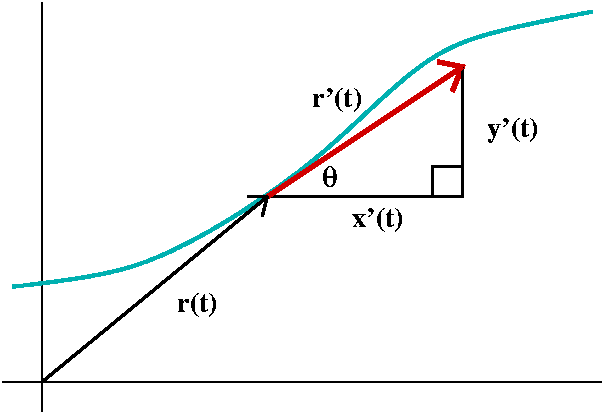Then I tried to analyze the idea of how a curve bends. Curvature will be a measure of this bending.(Today a plane curve, in R2, and tomorrow a space curve, in R3.) I began an analysis that was apparently first done by Euler in about 1750. A curve is a parametric curve, where a point's position at "time" t is given by a pair of functions, (x(t),y(t)). Equivalently, we study a position vector, r(t)=x(t)i+y(t)j. Here x(t) and y(t) will be functions which I will feel free to differentiate as much as I want.

There are some special test cases which I will want to keep in mind.

#1: a straight line
A straight line does NOT bend, so it should have curvature 0.

#2: a circle
A circle should have constant curvature, since each little piece of a circle of radius R>0 is congruent to each other little piece, and, in fact, the curvature should get large with R gets small (R is positive), and should get small when R gets large (and looks more like a line locally).

#3: "the" parabola
Even y=x2 might be a good test to keep in mind, since there the curvature should be an even (symmetric with respect to the y-axis) function of x, and should be bell-shaped, with max at 0 and limits 0 as x goes to +/- infinity.

The problem of understanding or defining curvature is to somehow extract the geometric information from the parameterized curve. That is, if a particle moves faster, say, along a curve, it might seem like the same curve bends more. So what can we do? Somehow the geometry of the curve should be separated from the dynamics (kinetics?) of motion along the curve.

We looked at theta, the angle that the velocity vector r´(t) makes with respect to the x-axis. How does theta change? After some discussion it was suggested that we look at the rate of change with respect to arclength along the curve: that is the same as asking for the rate of change with respect to travel along the curve at unit speed, and therefore somehow the kinetic information will not intrude on the geometry. This is the same as asking a bug to travel along the curve at unit speed, and to describe the rate of change of theta (the turning of r´(t), the velocity vector) as the bug strolls along the curve.

The quantity s and the quantity t
Arc length, s, on a curve is computable with a definite integral: sqrt(x´2+y´2) integrated from t0 to t with dt is the arc length. This is rarely exactly computable with antidifferentiation using the usual family of functions. But ds/dt is just sqrt(x´2+y´2) by the Fundamental Theorem of Calculus. And the Chain Rule suggests that
d*/ds(ds/dt)=d*/dt if * is any quantity of interest, such as theta. So to get d*/ds from d*/dt, multiply the latter by 1/(ds/dt) which is 1/sqrt(x´2+y´2).

By drawing a triangle we see that theta is arctan of y´/x´: theta=arctan(y´/x´). We can find d(theta)/dt: it is (y´´x´-x´´y´)/(x´2+y2)2. This uses the formula for the derivative of arctan, the Chain Rule, and the quotient rule. Then the previous results say that

d(theta)/ds=(y´´x´-x´´y´)/(x´2+y´2)3/2, a complicated formula.
This is what's called curvature, k (the Greek letter kappa).

Back to the test cases
 The straight line We compute for some constants A and B and C and D:x(t)=At+B x´(t)=A x´´(t)=0 y(t)=Ct+D y´(t)=C y´´(t)=0 so that the top of the curvature formula is 0. kappa=0 for any straight line.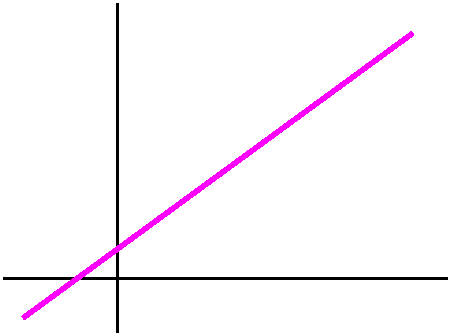A circle Suppose we have a circle of radius R centered at the origin. Then parametric equations are not too difficult to get:x(t)=Rcos(t) x´(t)=-Rsin(t) x´´(t)=-Rcos(t) y(t)=Rsin(t) y´(t)=Rcos(t) y´´(t)=-Rsin(t) Let me look first at the bottom of the curvature formula. This is (x´2+y´2)3/2. Notice that x´2+y´2=R2([-sin(t)]2+[cos(t)]2)=R2, so the 3/2´s power is R3. The top is y´´x´-x´´y´ and this is -Rcos(t)(-Rsin(t))--Rcos(t)Rcos(t)=R2·1. The whole curvature formula is then R2/R3 which is 1/R. When R is large, this is near 0. When R is close to 0 and positive, this is large positive. And the curvature is constant which it should be since the circle has the same local geometry at every point.A parabola Here I want to look at y=x2. A simple parameterization is good. So let's try:x(t)=t x´(t)=1 x´´(t)=0 y(t)=t2 y´(t)=2t y´´(t)=2 Now the bottom of kappa, (x´2+y´2)3/2, becomes (1+4t2)3/2, and the top, y´´x´-x´´y´, is just 2. So k=2/(1+4t2)3/2. Here the "local geometry" definitely changes from point-to-point. The most curvy (?) part of the graph is at the origin. As x-->+/-infinity, although the graph gets steeper and steeper, the curve locally actually gets more and more flat: the curvature-->0.The smaller figure is supposed to be the cruvature of the parabla.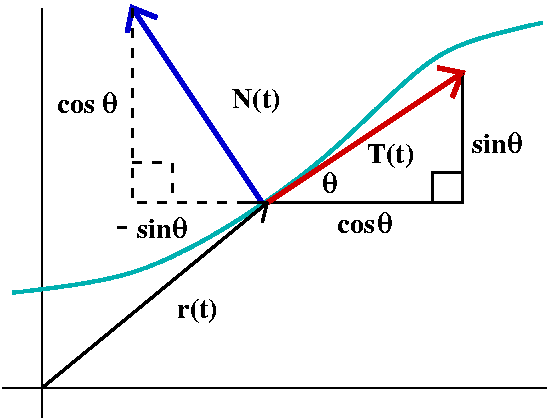I defined the unit tangent vector, T, to be a unit vector in the direction of r´(t). If theta is the angle that r´(t) makes with the positive x-axis, then T must be cos(theta)i+sin(theta)j. Also r´(t)=(ds/dt)T, where ds/dt is the length of r´(t), the speed. Now differentiate the formula for r´(t) using one of the product rules we had stated earlier. The result is r´´(t)=(d2s/dt2)T+ (ds/dt)d/dt(T). But T is cos(theta)i+sin(theta)j, and differentiation with respect to t is the same as differentiation with respect to s multiplied by ds/dt. But differentiation with respect to s gives (-sin(theta)i+cos(theta)j) multiplied by the derivative of theta with respect to s, and this is k. All this put together is:

r´´(t)= (d2s/dt2)T + k(ds/dt)2N
where N is (-sin(theta)i+cos(theta)j), a unit vector normal to T (check this by dot product!), where is called the unit normal.

We have decomposed acceleration into the normal and tangential directions. This can be significant physically. Here are two extreme situations.

Straight line motion: no transverse forces
I used this to show that motion in a straight line ( where k= 0) had no normal component, and therefore a particle moving in a straight line had no force needed transverse to its motion (remember force and acceleration are vectors related by the scalar m, according to Newton's second law).

Moving in a circle: always normal force
On the other hand, in our circular situation, the curvature was a positive number, and as long as the particle was moving (ds/dt not equal to 0) a force was needed to keep it on the circle. This is because the curvature k was non-zero, and so were the other terms. This is not at all "intuitively clear" to me.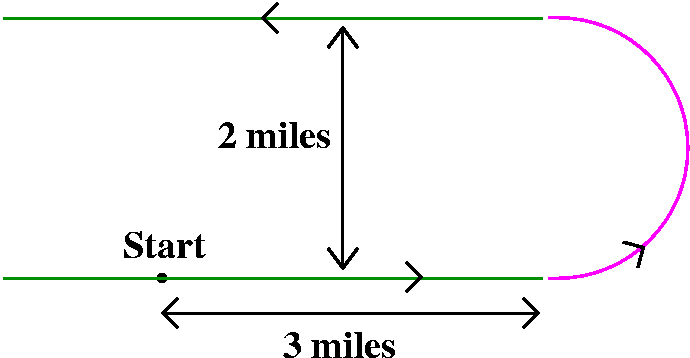QotD
A racetrack: this racetrack consists, as nearly as possible, of two straight line segments, each about 3 miles long, connected with a semicircular track. Since the racetracks were specified to be 2 miles apart, we "deduce" that the radius of the semicircle is 1 mile. The racetracks certainly have curvature=0, and the semicircle, which is Pi units long, has curvature 1. I asked that people try to draw the curvature of this racetrack. I remarked at the next class that students seem to have neglected the precise quantitative information that they were given.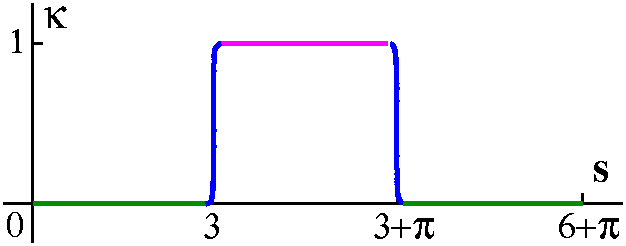I attempted to be "correct" both qualitatively and quantitatively in this graph. The blue curves are my attempts to draw smooth transitions between the curvatures of the circles.

Friday, January 27

Vector functions of a real number
Now the course moves on: we will deal with functions whose domain is all or part of R1 and whose range is R3. The motivation is really the motion of points in space, and the analysis of the resulting paths (curves).

Parametric curves: a nuisance?
So I began with some irritating examples.

1. The curve r(t)=<t,t,t>. This curve is a straight line. It goes through the origin, (0,0,0), and the path described is all of the line.
2. The curve r(t)=<sin(t),sin(t),sin(t)> is quite nasty psychologically if discussed immediately after the line. All of the coordinates are equal so every point on this curve is on the straight line. Some thought is needed to force yourself to agree that the "curve" is only a line segment from (-1,-1,-1) to (1,1,1) because sine's range is [-1,1].
3. Now the curve r(t)=<t2,t2,t2> is not too surprizing. It is a half line. Dynamically this represents the motion of a point starting way out in the first octant, then coming in (slower and slower) to (0,0,0). Then the point turns around (?) and starts out into the octant again, retracing its path and going faster and faster.
4. The curve r(t)=<t3,t3,t3> is all of the straight line, but the kinetic aspect is very different. Motion is not uniform, and gets faster as |t| gets larger.

Distinguishing between geometric aspects of the path of a particle and the dynamic aspects of the particle will take up most of our next class. This won't be obvious.

Pictures of a point in motion
Twisted cubic
The twisted cubic is the curve r(t)=<t,t2,t3>. It is one of the beginning curves shown to students in this subject, with the warning that here is how pictures can be deceptive. Here is a Maple command I used, and then I rotated the image and exported the pictures below.
spacecurve(<t,t^2,t^3>,t=-5..5,color=black,axes=normal,thickness=2);

 The view from the z-axis Here we look down on the curve from high up on the z-axis. This has the effect of suppressing (?) or deleting the z-coordinate from the triple, and we just see the geometry of the first two coordinates: (t,t2). Of course, this is the parabola y=x2.The view from the y-axis Now delete the central variable. In the xz-plane, the curve is the collection of points (t,t3) and this is z=x3, which is, I hope, a fairly familiar cubic. And, indeed, if you orient the twisted cubic properly, then Maple shows you the displayed picture.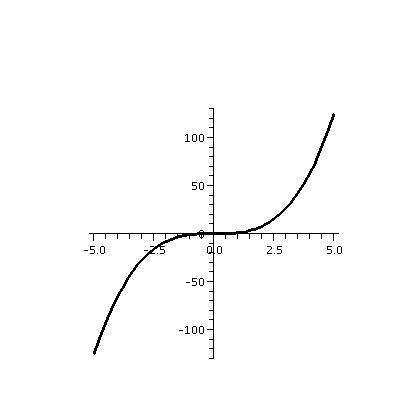The view from the x-axis This is picture which makes life difficult. Projected only the yz-plane, the collection of points (t2,t3) is the same as those points satisfying y3=z2. Now since z2 must be non-negative, any y's on this curve had better be in the right half of the yz-plane. And for each y>0, there are two z's (the positive and negative square roots). But the worst part is the behavior at the origin. z=+/-y3/2 has a horizontal tangent at (0,0): the curve has what's known as a cusp at the origin, a type of corner.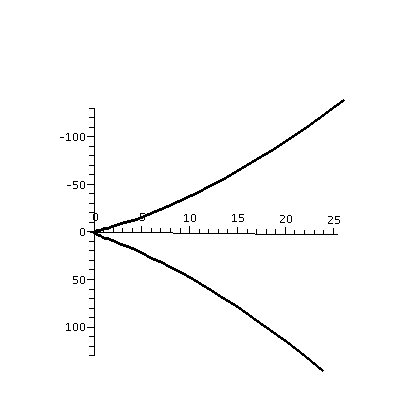An oblique view The students and I discussed at length whether this "curve" actually does have a corner in three-dimensions. The curve does not, and the pictures do not make this easy to see. To the right is sort of an oblique view of the curve as drawn by Maple. Although this picture seems to show a loop, the curve does not actually have any loops. The loopy look is a result of the angle I picked. Frequently, similar difficulties have occurred in dissections, when slides of different cells are prepared. Depending on the angles of the slices, very different pictures are seen.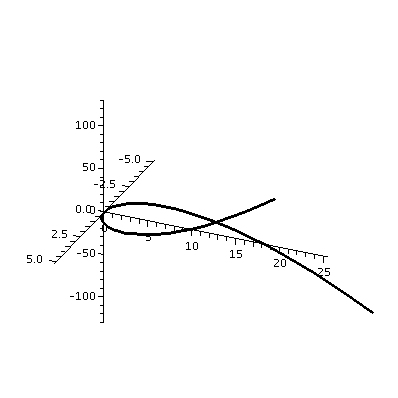Match up curves and formulas
We discussed the pictures and formulas which are in problems 19-24 of section 13.1. I would like to strengthen students' intuition associating illustrations of curves and their algebraic descriptions. While I do think that part of this might be innate talent, I think that far more of the intuition is a result of studying many pictures and many formulas. Students should be encouraged to do this.

Robots and molecules
I remarked that people who wanted to understand the conformation of biologically active macromolecules nned to learn how to look at curves in R3. Also, people who want to describe motion of robot arms are necessarily interesting in this subject.

The derivative
If r(t)=<a(t),b(t),c(t)> is a vector function of t, we can consider (1/h){r(t+h)-r(t)}. Inside the {} is a difference of vectors, and that's therefore a vector. Then multiplying by 1/h: that's a scalar multiple. So the result is a vector. It may happen that the limit as h-->0 of this quotient may or may not exist. If it does, we'll say that r(t) is differentiable and that the limit, which is labeled r´(t), will be called the derivative. It is also called the velocity vector.

It doesn't take many examples to convince yourself that the (vector) derivative will exist exactly when all of the scalar functions a(t) and b(t) and c(t) are differentiable, and that the components of the resulting vector derivative will be the derivatives of these functions.

Various formulas, especially product formulas
The derivative of u(t)·v(t) is u´(t)·v(t)+u(t)·v´(t). The derivative of u(t)xv(t) is u´(t)xv(t)+u(t)xv´(t). And there's even another product rule (multiplying a vector function by a scalar function and then differentiating). Since the cross product is not commutative, the order is important (we'll see an example next time).

Computations
I did some examples using leftovers from the textbook problems.

Meaning of the derivative
The derivative balances out a large scalar, 1/h (when h is small), with a very small secant vector, r(t+h)-r(t). That the limit exists is rather neat, and one should really not expect it!

The magnitude: speed
The magnitude of the velocity vector, |r´(t)|, is the speed.

The direction: unit tangent vector
The direction of r´(t)is gotten by taking r´(t) and dividing by its norm, |r´(t)|. This is a unit vector, called the unit tangent vecotr, and usually written T(t).

Velocity vector, tangent vector
So the direction of the velocity vector to tangent to the path of the particle, and its length represents the speed of the particle.

Tangent line to a helix
Look at the curve r(t)=<3cos(2t),3sin(2t),8t>. What is this?
The first two variables describe uniform circular motion. The radius of the circle is 3, and that's the distance from the central axis of this curve, which turns out to be a helix. The 2 changes the angular velocity of the curve, and doubles it. The 8 affects the "pitch", the angle of the helix, and also the distance between "loops". When 2t changes by 2Pi, the curve passes around one loop. That means the change in t is Pi, so the change in z is 8Pi.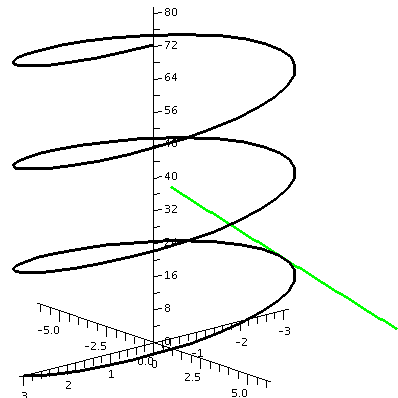Let's find the parametric equations of a line tangent to this helix when t=Pi/2. The line must pass through r(2)=<3cos(2[pi/2]),3sin(2[Pi/2]),8[Pi/2]>=<-3,0,4Pi>. A vector in the tangent direction can be gotten from the velocity vector. So: r´(t)=<-6sin(2t),6cos(2t),8> which, when t=Pi/2, gives <0,-6,8>. Therefore the parametric equations for the line are:
x=-3+0t
y=0-6t
z=4Pi+8t
To the right is a picture of both the helix and the tangent line just specified. And I only had to do it twice because I made a mistake.

Question of the day
I asked students to find the parametric equations of a line tangent to r(t)=<t2,1/t2,-4> when t=2.

Tuesday, January 24

Is your lecturer a mammal or a reptile?
101o Fahrenheit is about the same as 30.67o Réaumur. This is another temperature scale. See
here, for example.

Old QotD
I looked at last week's QotD's and then I computed a dot product and a cross product.
v=(1/sqrt{3})i-(2/sqrt{3})j+(5/sqrt{3})k and w=7sqrt{3}i-4sqrt{3}j+sqrt{3}k.

|     i          j         k     |
Then vxw=det| 1/sqrt{3} -2/sqrt{3} 5/sqrt{3} |
|  7sqrt{3} -4sqrt{3}   sqrt{3}  |
This is:
[(-2/sqrt{3})(sqrt{3})-5/sqrt{3}(-4sqrt{3})]i -[1/sqrt{3}(sqrt{3})-5/sqrt{3}(7sqrt{3})]j +[(1/sqrt{3})(-4sqrt{3})-(-2/sqrt{3})7sqrt{3}]k and this is 18i+34j+1ok (I hope I've gotten this correct -- it is more difficult when there is no one "watching".)
The cross product of two vectors is a vector. The signs involving the cross product can be very confusing.
v·w=(1/sqrt{3})(7sqrt{3})-2/sqrt{3}(-4sqrt{3})+5/sqrt{3}(sqrt{3})=20. The dot product of two vectors is a scalar.

Maple information
I then remarked that everyone who was a registered student and who had filled out a student information form should have gotten information about the first Maple assignment. Students who did not get that information should send me e-mail immediately. The due date for this assignment is Wednesday, February 1 and late work will not be accepted.

I will have office hours probably Tuesday and Thursday afternoons, but not today, since I need to go home and go to bed with my cold. You can see me in my office (Hill 542) on Thursday afternoon from 2:30 to 4:30. By then I might be healthier.Algebraic descriptions of lines and planes

Mostly I think I know what a line is and what a plane is. Here is a picture with one of both of them displayed. But what happens if we have severalof them, and we want to deal very precisely with them? It is useful, then, to have algebraic ways of describing and computing with lines and planes.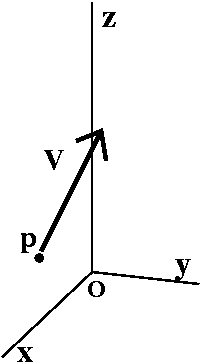Specifying a line
The following information will usually be the most convenient way to specify a line in this course:

• A point on the line (I'll call it p)
• A vector in the direction of the line (I'll call it v)
Then the line will be everything starting out at p going forward or backward in the direction of v. More precisely, if q=(x,y,z), then q will be on the line if the vector pq (from p to q) is a scalar multiple of v. This might be easier to describe with an example.

Example
If p=(3,9,5) and v=<4,-7,6>, then the vector pq will be . Suppose t is a scalar. The vector equation pq=tv works out to the following three scalar equations:
x=3+4t
y=9+-7t
z=5+6t
So these are parametric equations for the straight line determined by the data p and v. Notice where the 3 chunks of p's information go (no t's!) and where the 3 chunks of v's information (the direction) go (with the t's).

Other parametric representations
I then played with these equations for a while. For example, the equations
x=3+12t
y=9+-21t
z=5+18t
represent the same line. I just multiplied the direction v by 3. This doesn't change the actual geometric line which is parameterized by the equations: every point in "this" line is in the other line, and, similarly, the other way around. And
x=3-4t
y=9+7t
z=5-6t
represents also the same line: I multiplied the direction by -1 but still we will get the same total collection of points: the same straight line. And, perhaps more complicated:
x=7+4t
y=-2+-7t
z=11+6t
This is also the same line. Notice, please, that the direction vector is the same, but what I have changed is the "base point", (7,-2,11). But if you consider the original parameterization, and put t=1 in those equations, we will get (7,2,11). So, in fact, the descriptions sort of "overlay" one another: the v's are the same, and the we've just happened to start the parameterizations at different points on the same line.

There is a plethora of different parameterizations for the same straight line.
Plethora officially means "A superabundance; an excess."

Symmetric equations
Some manipulation gives another way to represent the line.
x=3+4t becomes t=(x-3)/4
y=9+-7t becomes t=(y-9)/(-7)
z=5+6t becomes t=(z-5)/6
so that we have (x-3)/4=(y-9)/(-7)=(z-5)/6. Such a collection of equations is called the symmetric form of the line. As far as I know, we won't describe lines this way in this course.

Two points determine a line
The original work of Euclid is available, translated and illustrated, for free on the web. You may see here. This work has been done by Professor Joyce of Clark University. So, way back, thousands of years ago, Euclid decided that a line would be determined by two points. How can we use such information to get an algebraic description?

Example
Suppose p=(3,3,-9) and q=(-5,2,1). What are parametric equations for the line through the points p and q?
The vector pq=<-5-3,2-3,-5-(-9)>=<-8,-1,4> is a vector in the direction of the line. We could use either p or q (I'll use p here) for a "base point" for the line. So we get the following parametric equations for the line:
x=3+(-8)t
y=3+(-1)t
z=-9+(4)t
The colors are an effort to show where to put the data in the parametric formulas.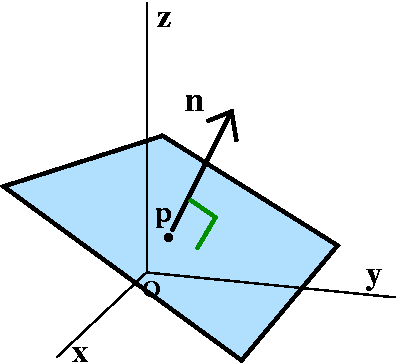Specifying a plane
In this course, the data specifying a plane will also turn out to be a point and a vector. What I'm about to describe may not look immediately useful, but it will be, really. So what we will need is

• A point on the plane (I'll call it p)
• A vector normal (perpendicular, orthogonal) to the plane (I'll call it n)
I hope that a picture can be persuasive. There are many planes which pass through the point p. But if you add on the requirement that, at the point p, the vector n be perpendicular, exactly one geometric "candidate" persists, and that's the plane I'd like to describe algebraically.

What condition(s) on x and y and z will guarantee that q=(x,y,z) is on the plane? Well, the vector pq should be perpendicular to n. We can check perpendicularity easily with dot product. An example may help.

Example
Suppose p=(4,5,-7) and n is the vector <11,2,31>. The vector pq is and pq is perpendicular to n edxactly when pq·n=0. And that is
11(x-4)+2(y-5)+31(z+7)=0
This is a fine equation. You don't need to "clean it up". Of course, it can be rewritten (if you must!) as
11x+2y+31z=44+10-217=163.

Again, there are many equivalent equations for one geometric plane since there are lots of vectors normal to one plane, and lots of points on one plane. And we can do the reverse:

Reversing ...
6x+-3y+7z=20 is the equation of a plane.
What is a vector normal to this plane? If you followed the previous discussion, I hope you can see that the components of such a vector are the coefficients of x and y and z in order. So n=<6,-3,7> is a vector normal to this plane.
What are all (non-zero) vectors normal to this plane? These vectors are the non-zero scalar multiples of <6,-3,7>.
What are the coordinates of a point on the plane 6x-3y+7z=20?
Part of the problem in answer this question is that there are so many possibly answers! For example, (0,0,20/7) is one answer, and so is (1,-7/3,1), and so is .... uhhh ... (1,000, -1,000, -8,980/7). There are many points on the plane.

Three points determine a plane
Again, Euclid declared that 3 points in space should determine a plane. So can we find an algebraic description of a plane through the points p=(3,3,-9) and q=(-5,2,1) and r=(4,2,2)? We need to find a vector n normal to the plane. Well, the vectors pq and (say) qr are in the plane's direction. The cross-product of these two vectors will be perpendicular to the plane. So: pq=<-8,-1,4> and qr=<9,0,1>, and

| i  j k |
det|-8 -1 4 |=-i-(-8-36)j-(-9)k=-i+44j+9k
| 9  0 1 |
is a normal vector. So -1(x-3)+44(y-3)+9(z-(-9))=0 is an equation of this plane. (We would get equivalent equations if we used q or r instead of p, or if we computed with pqxpr instead of pqxqr.)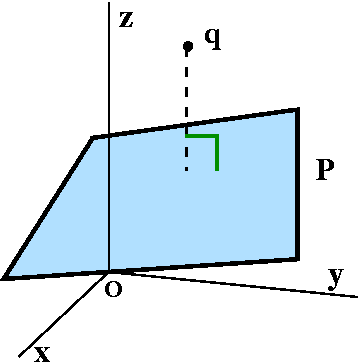Distance of a point to a plane
Suppose the point q is not on a plane, P. How can we find the distance from q to P? I did this in a rather clumsy way in class, mostly because I wanted to practice how to use line and plane equations. Let me start with specific information:
Suppose q=(2,1,3) and P is the plane specified by the equation 5x-7y+6z=10.
First, is q on the plane, P? Well, we can check this by substituting the coordinats of q into the equation presented for P: 5·2-7·1+6·3=10-7+18=21, and 21 is not 10, so q is not on P.
My strategy for finding the distance was to write the parametric equations for a line through q perpendicular to P, see where the line intersected P, and then compute the distance from q to that point. So let me try to carry this out.

• A line perpendicular to P through q Well, n=<5,-7,6> is a vector normal to P. So
x=2+5t
y=1-7t
z=3+6t
are parametric equations for the desired line.
• The intersection of the line and the plane Take the parametric equations and plug them into the equation for the line:
5x-7y+6z=10 becomes 5(2+5t)-7(1-7t)+6(3+6t)=10 which is (25+49+36)t=10-10+7-18 and this is 110t=-11 so t=-11/110=-1/10.
What does this value of t mean? It is the value of t which, when substituted in the parametric equations, gives the intersection point. So:
x=2+5(-1/10)=15/10
y=1-7(-1/10)=17/10
z=3+6(-1/10)=24/10
The intersection point is (15/10,17/10,24/10).
• The distance between q=(2,1,3) and the intersection point This is sqrt{(2-15/10)2+(1-17/10)2+(3-24/10)2}. This is sqrt(25+49+36)/10=sqrt(110)/10.

Another way ...
Several students had much better suggestions than the computation above. So here is another way: (2,1,3) is not on the plane 5x-7y+6z=10. I can find a point on the plane just by "guess" (as I did earlier, and I will guess for something convenient!): r=(2,0,0). Then the vector rq=<0,1,3> points from the plane to q. ow if I project rq onto the normal vector I will get a vector whose length is the distance from the plane to the point. This "projection" is just what I did last time when I found the parallel part of a vector. So n=<5,-7,6> is still a vector normal to the plane. So we just compute rq·n/|n|=(-7+18)/sqrt{110}=11/sqrt{110}, which is the same answer. This is more direct and more efficient.

QotD
Given a point and a vector, I asked students for parametric equations of a line in the direction of the vector, and for the equation of a plane through the point normal to the vector.

The following material was not done in class, maybe due to the instructor barely being alive.

Parallel lines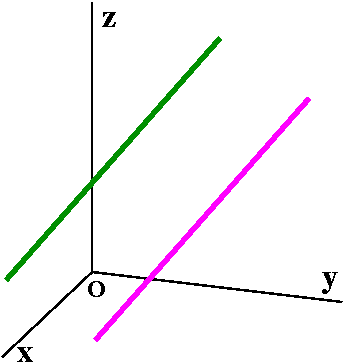I guess two lines are parallel if they are distinct (that is, separate lines!) and if their directions are non-zero multiples of one another. So, for example, the lines

Line A    Line B

x=5+t     x=30+t
y=3+2t    y=-9+2t
z=-1+3t   z=-1+3t
are parallel. Well, certainly their directions are multiples of one another (the multiple is 1). But why are they "distinct"? The point (5,3,-1) is on the first line. For it to be on the second line, uhhh ... 30+t=5, so t=-25. The second equation is then y=-9-(2)25=-59, and this is not 3. So (5,3,-1) is not on the other line.
These are parallel lines.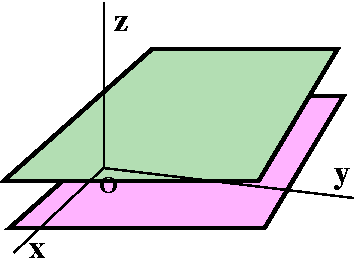Parallel planes
Two planes are parallel if they are distinct (that is, separate planes!) and their normal vectors are non-zero multiples of one another. For example, 2x+3y-z=56 and 4x+6y-2z=10 are parallel. Double the first equation and get the right-hand side of the second: that shows their normal vectors are non-zero multiples (the multiple is 2). But since twice 56=112 is not the same as 10, these planes are definitely distinct: different.

Skew linesAs dimensions increase, generally geometry gets more complicated. One phenomenon which does not occur in two dimensions is skew lines. A pair of lines is skew if they are not parallel and also do not intersect. So look at, say, the x-axis (where parametric equations could be x=t,y=0,z=0) and a line through (0,1,0) which is tilted with respect to the x-axis: x=t,y=1,z=t. These lines don't intersect since no point with second corrdinate 0 is on the second line. They aren't parallel since <1,0,0>, the direction of the first line, is not a non-zero scalar multiple of <1,0,1>. So they are skew.
Things get even worse as n, the dimension, increases. For example, it is possible to have pairs of skew planes (two dimensional objects) in R4.

Intersection of two planes
The two planes 3x-y=4 and 2x-2y+z=1 are not parallel. They intersect in a line. How can we get parametric equations for this line?

The planes are not parallel because the normal vectors (<3,-1,0> and <2,-2,1>, respectively) are not scalar multiples of one another. A vector in the direction of the line will be perpendicular to both of these normal vectors. The cross product gives us such a vector: <3,-1,0>x<2,-2,1> is -i-3j+4k. We also need a point on both planes. Well, if z=0 then we must find x and y which satisfy both 3x-y=4 and 2x-2y+0=1. I can do this: double the first equation and subtract the second equation. The result is 4x=7, so x=7/4 and then the first equation becomes 3(7/4)-y=4 so y=5/4. And (7/4,5/4,0) is a point on the line. Therefore a set of parametric equations for the line is
x=7/4+-t
y=5/4+-3t
z=0+4t.

Parametric equations for a plane
Another way to describe a plane is parametrically. Pick a point on the plane, and pick two vectors with distinct directions pointing in the plane. Wait: let's look at an example. Suppose the plane contains the point p=(9,-5,1) and the vectors u=<3,-2,5> and v=<6,5,4> point along the plane. Then for all numbers s and t, the points described by (9,-5,1)+su+tv describe a plane. I can break this up into components:
x=9+3s+6t
y=-5-2s+5t
z=1+5s+4t
We will deal with this alternative algebraic description of planes later when we discuss parametric surfaces. We can get back a vector normal to the plane by taking the cross product of u and v.

Friday, January 19

Maple thanks
I thanked the students who had shown up for the Maple labs. I don't think you need to fall in love with Maple, but you should see it as another tool you can use to learn.

What's on the web and why?
I discussed the material I would place on the web for this course. I called attention to
the list of students. I urged in class and I urge readers now to consider forming a study group for Math 251: do homework problems together, prepare for exams, etc. There is considerable evidence that students who work in study groups learn more and get higher grades.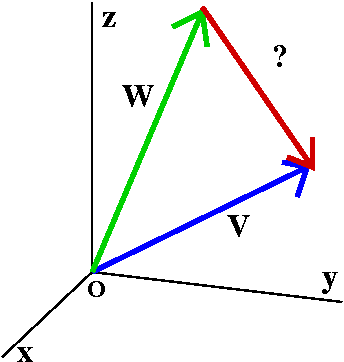Looking at lengths again
I started with v=ai+bj+ck so that |v|=srqt{a2+b2+c2}. If w=di+ej+fk, then |w|=srqt{d2+e2+f2}. If I draw the vectors v and w with their tails on the origin, then a vector, identified in the picture with "?", completes a triangle, going from the head of w to the head of v. Well, that vector isn't too mysterious. I know that w+?=v, so ?=v-w. In terms of components, we can write v-w=(a-d)i+(b-e)j+(c-f)k. The length of v-w is sqrt{(a-d)2+(b-e)2+c-f)2}. We saw last time that the sum of the lengths is not the length of the sum.

Really the squares of the lengths
With all the square roots, maybe we should be looking at the squares of the lengths. Also the algebra will be easier!
Then |v|2=a2+b2+c2
and |w|2=d2+e2+f2
and |v-w|2=(a-d)2+(b-e)2+c-f)2.
We can "expand" the last term to get

The obstacle to making it work
Well, if you compare terms, the sum |v|2+|w|2 is the same as |v-w|2 except for -2ad-2be-2cf=-2(ad+be+cf). So computations with lengths and squares of lengths will have to include that "mess". Let me forget the -2, which isn't that essential.

The dot product and its basic properties
Here we'll define the dot product. This is also called the scalar or inner product.
If v=ai+bj+ck and w=di+ej+fk, then v·w=ad+be+cf. In some places, v·w is written <v,w>.
The dot product obeys the following rules.

1. If v and w are vectors then v·w is a scalar (in this course this means "a read number").
2. (Commutativity) v·w=w·v. (You can see this is correct by looking at the pieces of v·w and w·v. For example, ad is the same as da.)
3. (Linearity or distributivity of vector addition over scalar multiplication) (v1+v2)·w=(v1·w)+(v2·w). (Again, you can check that this is correct by doing algebra with the components. A similar statement is true about the second factor, sice dot product is commutative.)
4. v·0=0.
5. v·v=|v|2.

Example with a little table
Suppose we have four rambunctious ("Boisterous and disorderly" vectors: v1 and v2 and w1 and w2. I also have this little multiplication table for their dot products:

· | w1 | w2
--------------
v1 | 3  | 7
--------------
v2 | 4  | -2
I asked students to compute something like
(4v1-3v2)·(5w1-2w2).
Here I expected people to distribute or take advantage of linearity on both the left- and right-had sides. So here is how the process might go:
(4v1)·(5w1-2w2)+(-3v2)·(5w1-2w2)
(4v1)·(5w1)+(4v1)·(-2w2)+(-3v2)·(5w1)+(-3v2)·(-2w2)
(4·5)v1·w1+(4·-2)v1·w2+(-3·5)v2·w1+(-3·-2)v2·w2
(4·5)(3)+(4·-2)7+(-3·5)(4)+(-3·-2)(-2)
And the answer seems to be .... 60-56-60-12=-68. I think this is correct. I make more errors in such computations when no one is watching me.

The law of cosines lurks ...
There's a formula which generalizes the Pythagorean Theorem's formula. For the triangle shown, here it is:
|v-w|2=|v|2+|w|2-2|v| |w|cos(theta)
Here theta is the angle opposite the side v-w. If theta is Pi/2, the cosine term is 0 and we get back Pythagoras. Here is the geometric view of the areas of the squares on the sides of a triangle.

The geometry of the dot product
Since we know that |v-w|2=|v|2+|w|2-2|v| |w|cos(theta) and we know that |v-w|2=a2-2ad+d2+b2-2be+e2+c2-2cd+e2=|v|2+|w|2-2v·w. We see that (cancelling the -2's)
v·w=|v| |w|cos(theta) where theta is the angle between v and w.
Notice that we can now see if v and w are not 0, then v and w are orthogonal (perpendicular, normal) exactly when v·w=0.

Determining an angle
Suppose v=<3,2,-1> and w=<5,2,6>. What is the angle between v and w?
Well, v·w=15+4-6=13, |v|=sqrt(14), and |w|=sqrt(65). Therefore cos(theta)=13/[sqrt(14)sqrt(65)]. This gives approximately (calculator-assisted computation here!) 64.55 degrees or (better, I guess) 1.126 radians.

Resolving a vector into perpendicular and parallel parts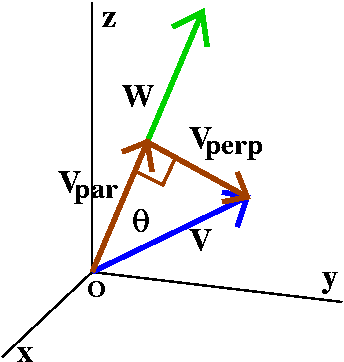Let me continue using the v=<3,2,-1> and w=<5,2,6> from the previous question. I'd like to show you how to write v as a sum of two vectors, vpar and vperp where vperp is perpendicular to w and vpar is parallel to w. This will be useful several times in the course, and is also important in physics.

How long is vpar? If you look at the picture, the vpar is part of a right triangle. It is the adjacent leg and v is the hypoteneuse. Theta is the angle between them. Therefore |vpar|=|v|cos(theta)=v·w/|w|. We did some of these computations just above, so |vpar|=13/sqrt(65). How can we get the correct direct? So we want a vector whose length is 13/sqrt(65) and whose direction is the direction of w. Well, we can create a unit vector (a vector of length 1) in the direction of w: that will be w/|w| which is <5,2,6>/sqrt(65). And now we adjust this unit vector by stretching it, to get [13/sqrt(65)]<5,2,6>/sqrt(65). This is about all I did in class, but let me finish things here. The vector is (13/65)<5,2,6>=<1,26/65,78/65>. Since v=vpar+vperp, we know that vperp=v-vpar=<3,2,-1>-<1,26/65,78/65>=<2,104/65,-143/65>.

Well, vperp should be perpendicular to w. So let's compute vperp·w=<2,104/65,-143/65>·<5,2,6>=10+(208/65)-(859/65)=(650+208-858)/65=(858-858)/65=0, so these vectors are indeed orthogonal.
Comment I admit (informative to you and irritating to me) that I did all these computations by hand, and I had to do them three times to get them to come out correctly.

Another product, introduced geometrically
I defined the dot product by a rather simple algebraic formula. The cross product has a complicated algebraic formula, and maybe it is easier to beguin by defining it geometrically.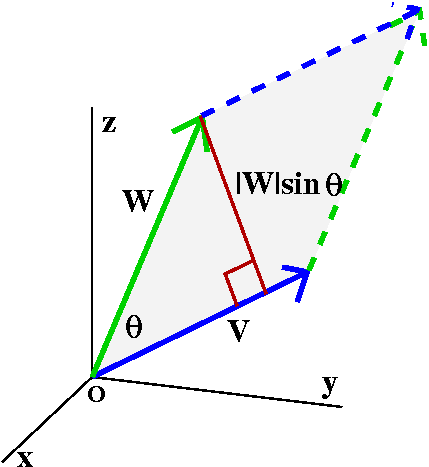The cross product (also called the vector or outer product) takes two vectors, v and w, and produces vxw, another vector. Since this is a vector, it is specified by its magnitude and direction.
Magnitude of vxw
Look at the the vectors v and w. There is a parallelogram which has sides v and w. The area (a non-negative quantity) of that parallelogram is the magnitude of vxw.
I forgot to say in class that there is an easy formula for the area in terms of the lengths of the vectors and the angle between them. The area is |v| |w|sin(theta). You get this by mulitplying the base by the altitude in the plane of the parellelogram.

Direction of vxw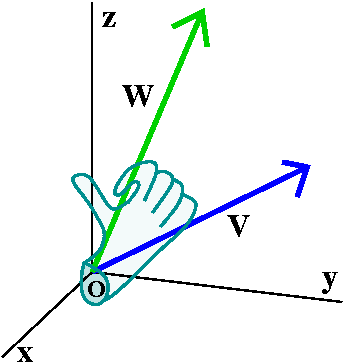Take your right hand and extend your thumb. Curl up your fingers. Insert your hand (I guess by though, not physically) so that your fingers go from v to w. Then your thumb will be (sort of) pointed perpendicular to the plane containing v and w. That perpendicular direction is the direction of vxw.

The only reason that such a weird product would be considered is that it is useful. We will see some geometric uses of it in this course and most of you will see uses in mechanics (torque), eletromagnetism, etc.

The i,j,k multiplication table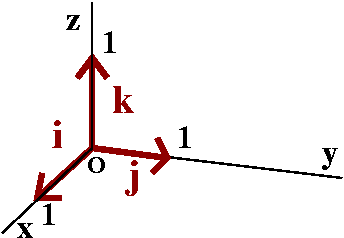So before anyone could yell too much I computed the cross product "multiplication table" for i and j and k. This wasn't too hard, although getting the directions correct involved some thought and some physical contortions. These are unit vectors and all mutually perpendicular. If the vectors in the product are the same, then the parallelogram involved collapses to a line segment and has no area. If the vectors are different, then the parallelgram is a square with 1 unit sides, and has area 1. The important thing is the sign of the answer and its direction. I tried to get that correct. If you were not in class or you were confused, I urge you to try to get some of the entries in this table yourself.

x |  i  |  j  |  k
--------------------
i |  0  |  k  | -j
--------------------
j | -k  |  0  |  i
--------------------
k |  j  | -i  |  0

Weird stuff
Things to notice:
ixj=k and jxi=-k. The cross product is not necessarily commutative.
(ixj)xj=kxj=-1 and ix(jxj)=ix0=0. The cross product is not necessarily associative.
This means that you can't necessarily rearrange or regroup cross products. This is annoying. The cross product does not resemble many other products you have seen.

Then the cross product in terms of components is ...
I then did the following awful computation, which you should never do:
If v=ai+bj+ck and w=di+ej+fk, then vxw=
I filled in all the values from the multiplication table above and got the following mess:

This last formula can be expressed in a very neat way if you know about determinants. It turns out that

| i j k |
vxw=det| a b c |
| d e f |
There's a very brief discussion of determinants in the textbook. I will probably evaluate this determinant most of the time by "expanding along the first row" but you may use any valid method.

Why is what I've done correct?
The definition I gave for cross product was geometric. Some of the properties of cross product are easy from the geometric definition. But I've just used linearity (or distributed the sum over the cross product), something like this: (v1+v2)xw=(v1xw)+(v2xw). Why is this true? This is not clear (!) to me at all from the geometric definition. Here is a paper which shows why you can distribute using geometry. Figure 6 on page 8 of this paper is a picture which should convince you that cross product does have the desired algebraic property. I hope you will find it convincing.

QotD
I gave two vectors (something like v=<3,1,-2> and w=<1,-2,4> and asked people to compute the dot and cross products of these vectors.

Torpor

1. A state of mental or physical inactivity or insensibility.
2. Lethargy; apathy.
3. The dormant, inactive state of a hibernating or estivating animal.
Torque
1. The moment of a force; the measure of a force's tendency to produce torsion and rotation about an axis, equal to the vector product of the radius vector from the axis of rotation to the point of application of the force and the force vector.
2. A turning or twisting force.

Tuesday, January 17

What is this?
This is Math 251. The lectures are done by me, and I will attempt to give lectures with the same approximate content on first and third periods each Tuesday and Friday. The first period perhaps will be more spontaneous but also will have more errors both in content and in presentation. The third period is likely to be a bit more organized, but perhaps less lively. Sigh.

Who am I?
I'm a faculty member in the Rutgers Math Department. Much of my professional work has involved differential equations involving functions of more than one variable. I actually like the content of this course, and I may get almost incoherent with excitement and pleasure when describing some of the subject matter. I haven't taught this course in almost a decade. I have taught Math 421 several times in the last few years. Math 421 is required for several engineering degrees (Mechanical, Chemical, Biochem), and almost everything done in that course builds directly on the material of this course.

Who are you?
Most of
the students in these sections are engineering students. Others are from such disciplines as chemistry, computer science, mathematics, meteorology, and physics.

What will we study?
I tried to briefly discuss the background of the course. The link indicated has more information.

Then we went on to "review" analytic geometry of one and two and even three dimensions. I put the word in quotes because most students who have taken courses in applied science and engineering have already seen the material we will discuss in 3 or 4 other courses.

R1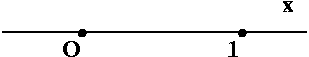This is supposed to be the real line. There's an origin and a unit length, and the conventional choice is to make the positive direction go off to the right. Each point on the line corresponds to a real number. The distance between points on the line which correspond to the real numbers a and b is defined to be |a-b|.

R2And here is the plane (I don't think I've ever heard anyone call it the "real plane"). The simplest situation is what's shown here. Two straight lines as axes, perpendicular to each. Every point corresponds to a unique ordered pair of real numbers. There is a set unit length on each coordinate axis, and almost always right is positive and up is positive. Later in the course we will consider situations where the coordinate axes are not necessarily perpendicular. And even where what corresponds to the axes are not straight. I hope that tilted axes could make sense if, for example, you were interested in looking at crystals which did not have rectangular symmetry.

R3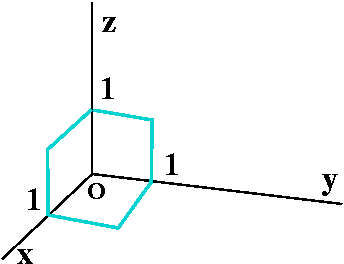In three dimensions, the geometric situation is specified by three mutually perpendicular lines. Points will correspond to ordered triples of real numbers. In almost every situation you are likely to encounter, these axes will be right-handed. Right-handed means ... means ... means ... well, it means what I sketched to the right (heh heh). The three dimensional world is complicated, and you will see that the choice of right-handedness has some interesting consequences.

{Levero|dextro} Here is a short discussion of parity in chemistry and physics. And here is a Wikipedia article on enantiomers, which are compounds that are non-superimposable mirror images of one another. This article is a bit more technical, but has many examples of pharmaceutical products which are mirror images.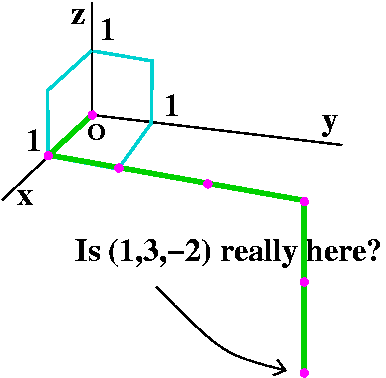Perilous pictures
Here is my attempt to draw the point with coordinates (3,2,-1). The green "path" with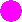's sort of tells how to find the point. I walk (?) from (0,0,0) to (1,0,0), then to (1,3,0), and finally to (1,3,-2). Eachis supposed to represent one unit of the path. I think that the complications of perspective and reducing the "object" from three dimensions to a two-dimensional representation may make even such a simple picture difficult to understand. If you weren't told that the point was supposed to be (1,3,-2), would you have guessed those were the coordinates? Sigh.
I will try to draw good pictures, but I won't always succeed. And even if I draw pictures which I believe are suitable, you may well not agree. Pictures are complicated.

 Some simple geometry and equations What points in R3 have y=2? Certainly (0,2,0), on the y-axis, is the only point on the y-axis with y=2. We can move up and down (changing z-coordinate) and sideways (changing x-coordinate). We can even change both. The collection of points we get is a plane, perpendicular to the y-axis through the point (0,2,0). An attempt at a picture is to the right. The words "Supposed" refer to the possibility that you don't agree it is a good picture! I mentioned that in this course I would probably regard the following as synonyms (the words mean the same) most of the time: perpendicular    orthogonal    normal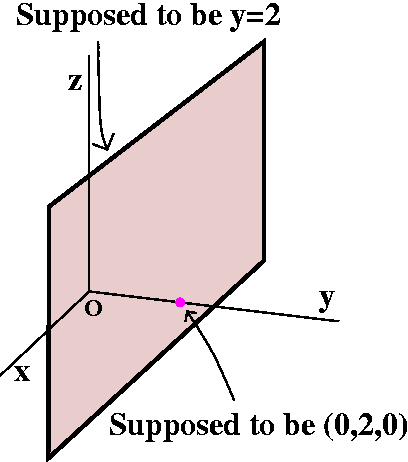What points in R3 have z<5? As one student observed, this is a half-space. The plane z=5 is the boundary of this region. It is a plane normal to the z-axis through the point (0,0,5). The plane, however, is not included in the region specified by z<5. The region does not include its boundary. A region which does not include its boundary is called an open region (we'll go into more detail later). So what I've attempted to show in the picture is an open half-space.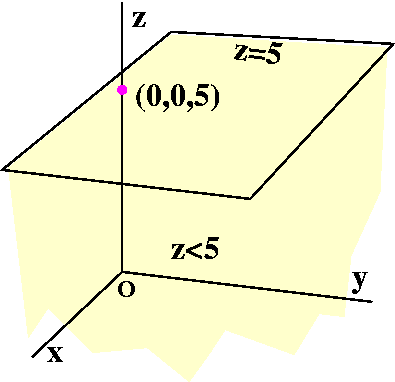A brick in space
 Let's imagine a brick in R3 with its sides parallel to the xy and yz and xz planes. Maybe this could be called a right-angled parallelopiped. Suppose one corner (also called a vertex) has coordinates (a,b,c) and the diagonally opposite corner has coordinates (d,e,f).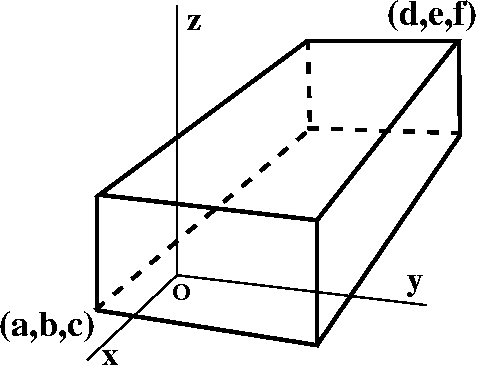Suppose now we look at the corner displayed in the accompanying picture, the corner illustrated with. What are the coordinates of that point? If you can "see" what the picture is supposed to show, then the designated corner is just moved "out" parallel to the direction of the y-axis. The x-coordinate doesn't change at all, and the height from the xy-plane (which is the z-coordinate) also doesn't change. Therefore the coordinates ofare certainly (a,?,c). What about the second coordinate of the point? If you look at the picture with me, you can see that the entire face or side of the solid betweenand the point (d,e,f) has constant y-value: it is perpendicular to the y-axis. Therefore the mystery middle coordinate must be the same as the middle coordinate of (d,e,f), and so=(a,e,c).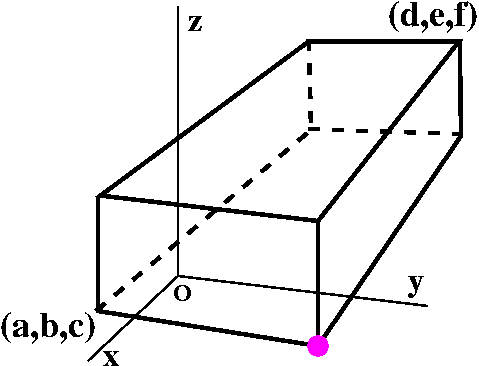Now let us play the same game with another vertex (or corner). So the designation ofhas been moved to the corner shown. What are the coordinates of this point? This point is on the same face or side of the solid so it certainly shares the second or y-coordinate with both (d,e,f) and (a,e,c), so=(?1,e,?2). Well, the third coordinate, ?2, must be c since the point we're looking at is certainly on the bottom face of the solid. The first coordinate might be the most puzzling, but both (d,e,f) andare on the "back" face (that is part of the plane x=d) of the solid, so the x-coordinate we're looking for is d. Therefore thisis (d,e,c). A good exercise for you is to figure out the coordinates of some of the other corners of the brick.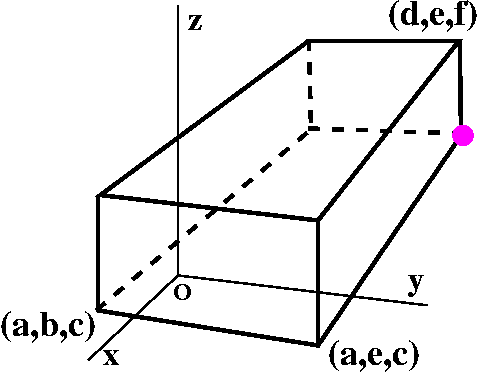Now I'd like to compute the length of some of the edges of the brick. For example, what is the length of the line segment connecting (a,b,c) and (a,e,c)? This is the distance between these two points. Notice that the only coordinate which is varying is the middle one. This side is a line segment which is parallel to the y-axis. We can measure length along it only by thinking about the one-dimensional distance between b and e. That distance is |b-e|. The reason for the absolute value signs is that distance is supposed to be non-negative.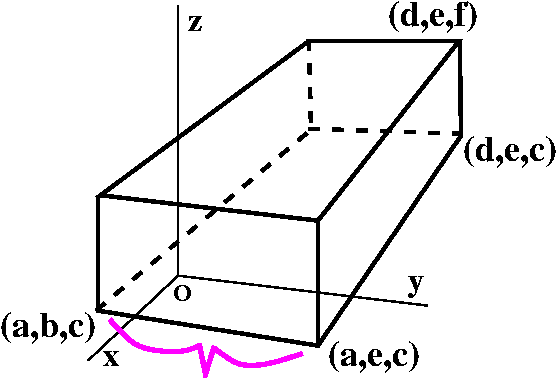We can try to do the same thing with the edge connecting the points with coordinates (a,e,c) and (d,e,c). Here the only coordinate difference is in the first, x-, coordinate. So distances again should be measured as if they were in one dimension, along the parallel x-axes. The distance we want is |a-d|.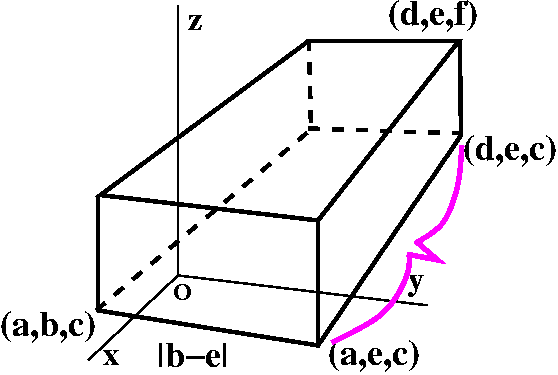Now we can be even more bold. (Well, bold for a math class.) We can find the distance from (a,b,c) to (d,e,c). Well, look carefully and see that there is a right triangle whose hypotenuse is the distance wanted, and whose "legs" have distances we already know. Then Pythagoras declares that the length of the hypotenuse is sqrt(|b-e|2+|a-d|2) If you are somewhat alert this result is not too surprising. The bottom face of this brick is where z=c. The formula written is just the ordinary two-dimensional distance between the points (a,b) and (d,e).I'm not going to try to put that formula in the picture. I will just label the distance Blah. Now I want the distance along one of the main diagonals of the brick, from (a,b,c) to (d,e,f). There is, as shown, another right triangle. The hypotenuse is the distance I want, and the two legs have distances we know: one is Blah and the other is ... the other is: well, the same ideas as before (since the endpoints differ in only one coordinate) tell me that the distance is |c-f|. Therefore (again Pythagorus) the distance between the farthest apart corners is sqrt(Blah2+|a-d|2) But let me write it out in all of its glory (or gory [details, that is!]):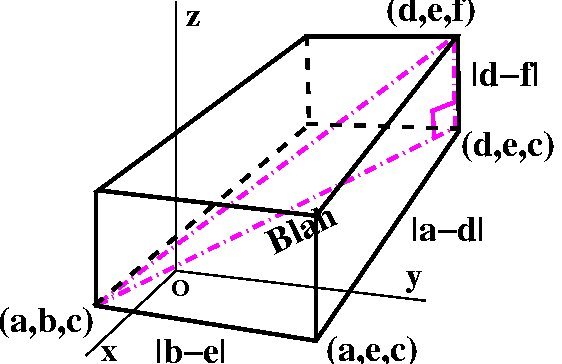sqrt({sqrt(|b-e|2+|a-d|2)}2+|a-d|2)
Here I inserted the formula we previously got for Blah. But lots of what is written in this formula is superfluous. The square of a square root is always the number. We don't need absolute values if we are square a number. So therefore we can make a spontaneous (!) definition:

The Euclidean distance
The (Euclidean) distance between (a,b,c) and (d,e,f) is sqrt((a-d)2+(b-e)2+(c-f)2).

A bunch of comments

• First, math definitions shouldn't (and generally aren't) written at random. They ideally should be supported by examples and "intuition". Here the word intuition really means looking at lots of situations and extracting the important common ideas.
• Second, the definition actually shows you how to define distance between points in R17, if you would ever want to. The definition should be something like the square root of the sum of the squares of the differences between the respective coordinates of the points. And this actually (for many purposes) is a good definition. The distance between two points is 0 exactly when the points are the same, and the distance between p and q is the same as the distance between q and p. Math majors who have taken Math 300 might recognize the skeleton of a definition using mathematical induction. But I can't talk about this here.
• I remark that the distance defined is called the Euclidean distance because there are other ways of defining "good" distances, and some of them are useful in other contexts. For example, students who study digital signal processing will find out that the Euclidean distance is perhaps not always correct when comparing signals. In this course, the Euclidean distance will be the only one used, so I will almost always omit the adjective "Euclidean". What is nice about the Euclidean distance, though, is that it is very computationally neat, and leads to the method of least squares, which is a very commonly used method of fitting "curves" (function descriptions) to data points.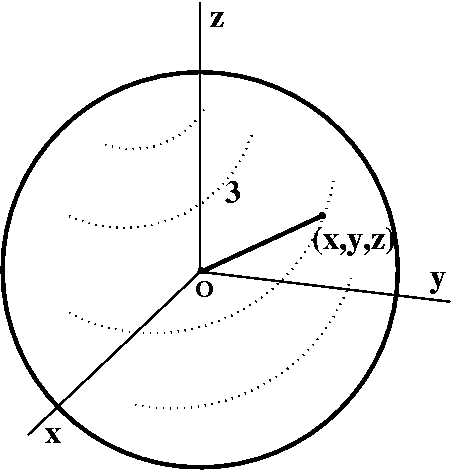A sphere of radius 3
What algebraic condition on a point (x,y,z) is equivalent to the geometric statement that the point lies on a sphere of radius 3 centered at (0,0,0)? This means that the distance from (x,y,z) to (0,0,0) should be 3, or sqrt((x-0)2+(y-0)2+(z-0)2)=3 which of course is the same as x2+y2+z2=9.

Completing the square to "recognize" a sphere
We could of course "reverse" the process if we're given an equation (or at least an equation of the correct form). For example, the equation
x2-3x+y2+4y+z2-7=0
represents a sphere. What is its center and its radius? The key algebraic maneuver here is completing the square, and everything works very much as in two dimensions.
x2-3x --> x2+2(-3/2)x --> x2+2(-3/2)x+(-3/2)2-(-3/2)2 --> (x-3/2)2-(-3/2)2
y2+4y --> y2+2(2y) --> y2+2(2y)+22-22 --> (y+2)2-22
We don't have any term with z to the first power. So the equation becomes:
(x-3/2)2-(-3/2)2+(y+2)2-22+z2-7=0
which is the same as
(x-3/2)2+(y+2)2+(z-0)2=(-3/2)2+22+7
and we can "read off" that the center is (3/2,-2,0) and the radius is sqrt((-3/2)2+22+7). It is easy to make mistakes with minus signs when identifying the center. It is also easy to forget to take the square root when identifying the radius. Oh well.
If we had different (but positive) numbers in front of the squares (x2 and y2 and z2) then we'd get an egg-shaped object, an ellipsoid. We'll see more about this later.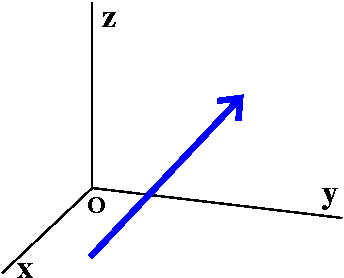Vectors
A vector is a directed line segment. It is used as a mathematical model for any quantity which has both magnitude and direction. For example, some of the following quantities are vectors and some are not:

• Odor I guess this is not a vector. Some students have no sense of humor.
• Velocity This is, of course, one of the basic examples of a vector. Here the length of the vector corresponds to speed: "I am traveling at 25 miles per hour" (in metric, I think that is 78 hectares per wombat). Of course the direction of the vector corresponds to the direction of motion: ""I am traveling at 25 miles per hour North by Northwest" (in the metric system, this direction is called "Colder").
• Force Another basic example of a vector. The magnitude is the amount of oomph in the force (I am giving up on units) while the direction is the direction the force is pushing in.
• Color Of course, this got some grudging laughter. Actually, though, if you are interested in colors displayed, for example, on a computer screen, colors are frequently specified as a vector of three integers in the range from 0 to 255. So this color is specified by the vector (0,255,153). Here the first number refers to the amount of Red, the second to the amount of Green, and the third, to the amount of Blue: this is the RGB triple. And actually sense can be made out of this as a vector.
• Etc. You will meet various vector quantities in this course and in other courses and work.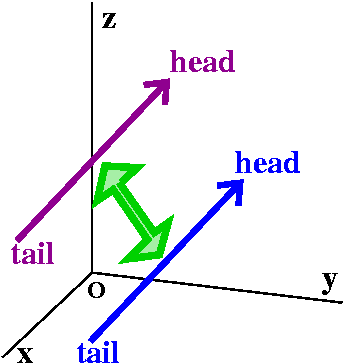Equal vectors
A vector can also be specified by its head and tail. Two vectors will be called equal (maybe the official word is equivalent) if when the tail of one of them is moved to the tail of the other, then their heads are in the same place.

Vectors are used to do algebra in more than one dimension. This is because algebra has really been successful, and the interaction between algebra and geometry has paid off well in both directions. Therefore we'll need to add and multiply vectors. Efforts to multiply run into serious difficulties, as we will see next time. Addition is neat and "everything" works well. As I mentioned in class, the generally accepted definition for vector addition models some situations which can be experienced and measured in "real life" with forces, velocities, etc. The word resultant is sometimes used in connection with this definition.

Definition of vector addition
 First suppose there are two vectors, v and w, which are roaming, free and happy in R3. We take the vector w and drag it so that the tail of w is at the same point as the head of v. The vector v+w is now defined using the geometric display we just arranged. v+w the vector whose tail is at the tail of v and whose head is at the head of w.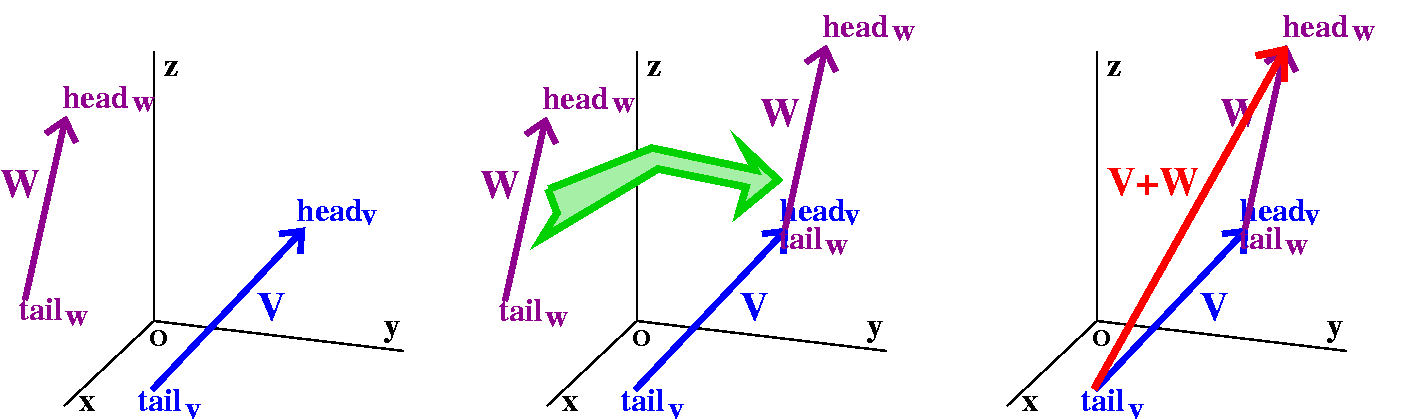Properties of vector addition

1. Reality Addition (superposition, resultant) of vector quantities in physical "reality" is modeled by vector addition.
2. Commutativity v+w=w+v for all pairs of vectors v and w.
This can be proved from the geometric definition by drawing a parallelogram which has one pair of sides translates of v and the other pair of sides translates of w. Anyway, the order in which you add vectors doesn't matter.
3. Associativity (v+w)+u=v+(w+u) for all triples of vectors v, w, and u.
I think this can be proved by drawing some parallelopided (three-dimensional analogue of a parallelogram) with sides gotten form v and w and u: but the diagram would almost be more annoying than just thinking about it. The important consequence is that you can group adding vectors in any way you want, and the result will be the same.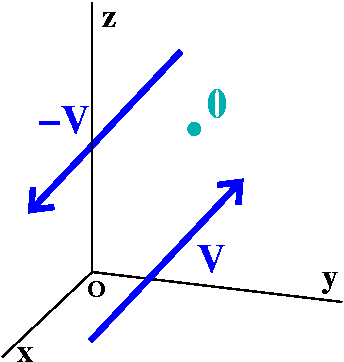Zero and minus
The zero vector has its head equal to its tail. If v is a vector, then -v is the vector whose length is v's but whose direction is the reverse: so the head of -v is the tail of v and the tail of -v is the head of v. Huh. Say that fast.
I am especially proud of the image of the zero vector in the picture to the right. I wanted to show the special beauty of the zero vector. I tried several different angles. (This is a joke!)

Then v+(-v)=0, and v+0=0 etc. for all vectors.

Scalar multiplication
In this course the scalars will be real numbers. Scalars are things that multiply vectors. Many of the students in the course will see later situations where the scalars are complex numbers. In the case of the RGB vectors above (and other situations which arise in computer science and electrical engineering) the scalars are much less familiar.

If c is a scalar and v is a vector, then the vector cv is defined by the following:
If c>0 the direction of the vector cv is the same as the direction of v, and the length of cv is the length of v multiplied by c.
If c=0 then cv is the zero vector.
If c<0 the direction of the vector cv is the same as the direction of -v and the length of cv is the length of v multiplied by |c|. (The absolute value makes sure that lengths stay positive.)
Here are pictures of v and -v and 3v and -2v.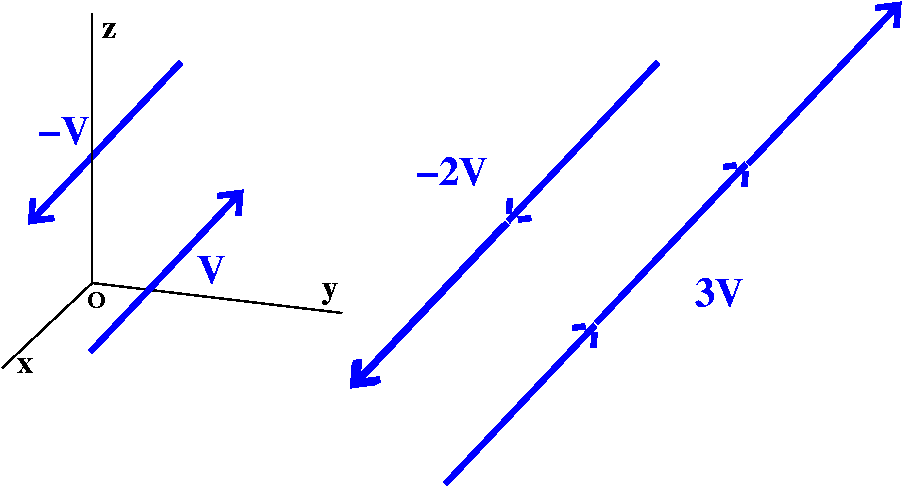Important vectors Some vectors are more important than others, especially if you have a coordinate system. The vectors i and j and k are vectors of length 1 (such vectors are called unit vectors) which are parallel to the coordinate axes x and y and z, respectively.Now take any vector v and move it so that the tail of v is at the origin, (0,0,0). Then the head of v will be at some point, (a,b,c). If you think about the geometry I hope you can convince yourself that v=ai+bj+ck. We have written v as a sum of its components. The textbook also uses the notation v= for this.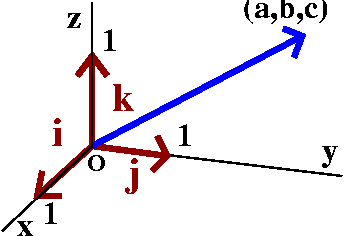Vector addition in terms of components
Since vector addition is commutative and associative, and certainly (c1+c2)(any vector)=(c1)(that vector)+(c2)(that vector), we see clearly that if v=ai+bj+ck and w=di+ej+fk, then v+w=(a+d)i+(b+e)j+(c+f)k.
The components of the sum are the sum of the respective components. If you know the components, it is easy to compute vector sums and scalar multiples of vectors.

The head and the tail produce the vector (algebraically)
Suppose the point P has coordinates (x1,y1,z1) and the point Q has coordinates (x2,y2,z2). Then the vector from P to Q (so P is the tail and Q is the head) is just (x2-x1)i+(y2-y1)j+(z2-z1)k. I hope that you can draw a picture convincing yourself of that, or you can look in the text.
Example The vector from P=(1,2,-3) to Q=(-5,6,2) is (-5-1)i+(6-2)j+(2-[-3])k=-6i+4j+5k. It is easy to make mistakes with signs in this computation.

How long is a vector?
I will use length and norm and magnitude to mean the same thing about a vector: its length. If v=ai+bj+ck, then we can think of the tail of v as sitting at (0,0,0) and the head of v, at (a,b,c). The length formula we got then says that the length must be sqrt(a2+b2+c2). So if you know the components, the magnitude can be computed. In the textbook we are using the magnitude is written |v|. Please note that in some books and some situations, this quantity is written ||v|| (with double "absolute value" bars).

NO! NO, NO, NO!!!
Suppose that v=<1,2,3> and w=<-2,3,2>. Then v+w=<-1,1,5>. And |v|=sqrt(14) (approximately 3.74), |w|=sqrt(17) (approximately 4.12), and |v+w|=sqrt(27) (approximately 5.19). In general, these numbers are not related at all. Please realize this.

Next time I will explore how the differences can be explained, as we discuss multiplication of vectors.

QotD
The Question of the Day asked: if v=<5,2,3> and w=3j-2k, what is 3v+2w?

Maintained by greenfie@math.rutgers.edu and last modified 1/19/2006.